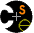Parallel ComputingSyllabusProgramming with ZPLZPL Guide (pdf)AssignmentsAssignment 1 (and soln)Assignment 2 (html) (pdf) (ppt)Assignment 3Assignment 4# Assignment 3

## Due Dec. 9 before class

Submit solution by email to Adam prior to class on Dec. 9

## Performance models and User defined reductions

The PSP paper gives two mode computations, which have been duplicated below for your convenience.
1. Use WYSIWYG analysis to say which is better
2. Create custom maxmode to improve last line
```-- Standard mode code
[1..n] begin
S := 0;
for i := 1 to n do
[i..n]     S += ((>>[i] V) = V);
end;
count := max<< S; -- largest freq count
mode := max<<((count = S) * V); -- get mode
end;

-- PSP mode code
[1,1..n] begin -- assume R = [1..n,1..n]
-- assume row 1 of V is input
[1..n,1]   Vt := V#[Index2,Index1]; -- transp
-- Replicate, compute and collapse
S := +<<[R] (>>[1,]V = >>[,1]Vt);
count := max<<  S;
mode := max<< ((count = S)*V);
end;

Hints: Reasoning is what counts in (1); in (2) use global data
reference

```Department of Computer Science & Engineering University of Washington Box 352350 Seattle, WA  98195-2350 (206) 543-1695 voice, (206) 543-2969 FAX [comments to carlson]